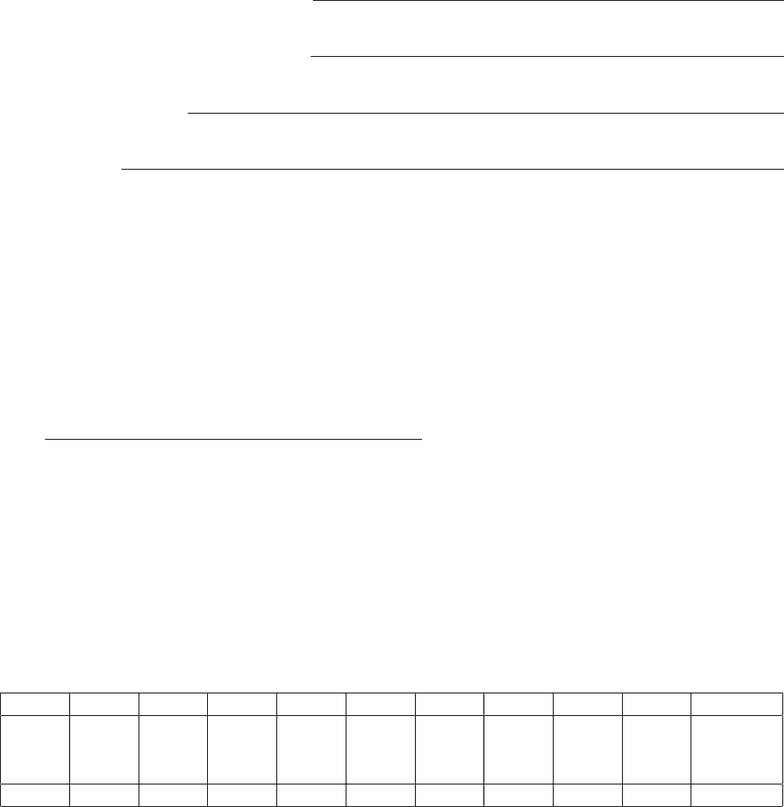Study Guides (390,000)
CA (150,000)
UTSC (10,000)
Mathematics (1,000)
MATA32H3 (100)
All (60)
Final

# MATA32H3 Final: MATA32H3 Final Exam 2015 FallExam

Department
Mathematics
Course Code
MATA32H3
Professor
All
Study Guide
Final

This preview shows pages 1-3. to view the full 12 pages of the document.*** Sorry...solutions will not be provided ***
University of Toronto at Scarborough
Department of Computer and Mathematical Sciences
FINAL EXAMINATION
MATA32F - Calculus for Management I
Examiners: R. Haslhofer Date: December 13, 2015
R. Grinnell Time: 2:00 pm
E. Moore Duration: 2 hours and 50 minutes
Provide the following information
LAST NAME (CAPITALIZE)
Given Name(s) (PRINT BIG)
Student Number
Signature
1. This examination has 12 numbered pages. At the beginning of the exam, check that all of
these pages are included.
2. If you need extra space for any question, use the back of a page or the blank page at the end
of the exam. Clearly indicate the location of your continuing work.
3. The following are forbidden at your work space: calculators, i-pads, smart/cell phones, all
other electronic devices (e.g. electronic translation/dictionary devices), extra paper, notes,
textbooks, opaque carrying cases, food, and drinks in paper cups/boxes or with a label.
4. You may write in pencil, pen, or other ink.
Do not write anything in the boxes below
1 2 3 4 5 6 7 8 9 10 TOTAL
16 15 16 15 14 11 16 17 14 16 150
1

Only pages 1-3 are available for preview. Some parts have been intentionally blurred.η=p/q
dp/dq S=P(1 + r)nS=R[(1 + r)n1
r]A=R[1(1 + r)n
r]
n
k=1
k=n(n+ 1)
2
n
k=1
k2=n(n+ 1)(2n+ 1)
6
Exam Instructions Write clear neat solutions in the answer spaces provided. Full points
will be awarded only if your solutions are correct, complete, and suﬃciently display appropriate
concepts and knowledge from MATA32F.
1. (a) Let f(x) = 2(5x). Calculate f(2)(1). [3 points]
(b) Let u(t) = 3t26tand u(1) = 4. Find u(4). [5 points]
(c) Assume Newton’s method is used to approximate the number 20 and let x1= 4.
Calculate x2. [5 points]
(d) Let an eﬀective rate be reand let rbe the APR compounded quarterly that is equivalent
to re. Solve for rin terms of re. [3 points]
2

Only pages 1-3 are available for preview. Some parts have been intentionally blurred.2. The parts of this question are independent of each other.
(a) Assume yis deﬁned implicitly as a function of xby the equation 2y+ln(xy2) = 1.
Solve for xwhen y= 1 in this equation, and then evaluate dy
dx at the point (x, 1).
[7 points]
(b) Let y=2x(x2)
x2+ 9 . Calculate the exact value of y(4) and state your answer as a
fraction in lowest terms. [8 points]
3